# Data Structures, Algorithms, & Applications in Java Pairing Heaps Copyright 1999 Sartaj Sahni

Introduction
Definition and Operations
isEmpty, size, and getMax
put
increaseKey
removeMax
remove
Implementation Considerations
Complexity and an Enhancement

Introduction
In the text, we studied two data structures--heaps and leftist trees--for the representation of a priority queue. When a max heap is used to represent a max priority queue, the `put` and `removeMax` operations take `O(log n)` time, where `n` is the number of elements in the priority queue. The remaining priority queue operations--`isEmpty`, `size`, and `getMax`--take `O(1)` time each. Leftist trees provide the same asymptotic complexity as do heaps. Additionally, when a leftist tree is used, two priority queues can be melded in `O(log n)` time.

In many applications, we are concerned more with the time it takes to perform a sequence of priority queue operations than we are with the time it takes to perform an individual operation. For example, when we sort using the heap sort method, we are concerned with the time it takes to complete the entire sort; not with the time it takes to remove the next element from the max heap. In these applications, it is adequate to use a data structure that has a good amortized complexity for each operation type. Fibonacci heaps and pairing heaps are two of the more popular priority queue data structures for which the amortized complexity of priority queue operations is good.

When a max Fibonacci heap is used, the actual and amortized complexities of various operations on an `n` element priority queue are

```
Operation        Actual Complexity         Amortized Complexity

isEmpty          O(1)                      O(1)
size             O(1)                      O(1)
getMax           O(1)                      O(1)
put              O(1)                      O(1)
removeMax        O(n)                      O(log n)
meld             O(1)                      O(1)
remove           O(n)                      O(log n)
increaseKey      O(n)                      O(1)

```

The operation `remove(theNode)` removes from the data structure an arbitrary node `theNode`, and the operation `increaseKey(newValue, theNode)` replaces the key (i.e., the priority) in the node `theNode` by a larger value `newValue` (for a min priority queue, the corresponding operation `decreaseKey(newValue, theNode)` replaces the key in the node `theNode` by a smaller value `newValue`).

When an auxiliary two pass max pairing heap is used, the actual and amortized complexities for the above operations are as below.

```
Operation        Actual Complexity         Amortized Complexity

isEmpty          O(1)                      O(1)
size             O(1)                      O(1)
getMax           O(1)                      O(1)
put              O(1)                      O(log n)
removeMax        O(n)                      O(log n)
meld             O(1)                      O(log n)
remove           O(n)                      O(log n)
increaseKey      O(1)                      O(log n)

```

Although the amortized complexities given above are not known to be tight (i.e., no one knows of an operation sequence whose run time actually grows logarithmically with the number of increase key operations (say)), it is known that the amortized complexity of the increase key operation is `Omega(log log n)`. The papers On the efficiency of pairing heaps and related data structures, by M. Fredman and Information theoretic implications for pairing heaps, by M. Fredman, ACM Symposium on the Theory of Computing, 1998 provide an information theoretic proof of this lower bound on the amortized complexity of the increase key operation for pairing heaps.

Experimental studies indicate that pairing heaps actually outperform Fibonacci heaps. Therefore, we develop pairing heaps only.

Definition and Operations
Pairing heaps come in two varieties--min pairing heaps and max pairing heaps. Min pairing heaps are used when we wish to represent a min priority queue, and max pairing heaps are used for max priority queues. In keeping with our discussion of heaps and leftist trees in the text, we explicitly discuss max pairing heaps here. Min pairing heaps are analogous.

A max pairing heap is simply a max tree (see Definition 9.1 of the text) in which the operations are performed in a manner to be specified later. Four max pairing heaps are shown below. Notice that a pairing heap need not be a binary tree.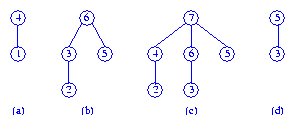isEmpty, size, and getMax
In a pairing heap, the `isEmpty` and `size` operations are done by maintaining a variable `size` which gives the number of elements currently in the data structure.

Since the max element is in the root of the max tree, the `getMax` operation is done by returning the element in the root.

Two max pairing heaps may be melded into a single max pairing heap by performing a compare-link operation. In a compare-link, the roots of the two max trees are compared and the max tree that has the smaller root is made the leftmost subtree of the other tree (ties are broken arbitrarily).

To meld the max trees (a) and (b), above, we compare the two roots. Since tree (a) has the smaller root, this tree becomes the leftmost subtree of tree (b). The resulting tree, tree (e), is shown below.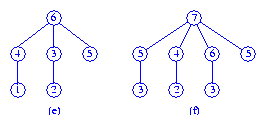Tree (f) is the result of melding the trees (c) and (d). When we meld the trees (e) and (f), the result is the tree (g).put
To put an element `theElement` into a pairing heap `p`, we first create a pairing heap `q` with the single element `theElement`, and then meld the two pairing heaps `p` and `q`.

increaseKey
Suppose we increase the key of the element in node `theNode`. When `theNode` is the root or when the new key in `theNode` is less than or equal to that in its parent, no additional work is to be done. However, when the new key in `theNode` is greater than that in its parent, the max tree property is violated and corrective action is to be taken. For example, if the key in the root of tree (c) is increased from `7` to `10`, or when the key in the leftmost child of the root of tree (c) is increased from `4` to `6` no additional work is necessary. However, when the key in the leftmost child of the root of tree (c) is increased from `4` to `9` the new value is larger than that in the root (see tree (h)) and corrective action is needed.Since pairing heaps are normally not implemented with a parent pointer, it is difficult to determine whether or not corrective action is needed following the key increase. Therefore, corrective action is taken regardless of whether or not it is needed except when `theNode` is the tree root. The corrective action consists of the following steps:
1. Remove the subtree with root `theNode` from the tree. This results in two max trees.
2. Meld the two max trees together.

Figure (i) shows the two max trees following step 1, and tree (j) is the result of step 2.

removeMax
The max element is in the root of the tree. When the root is removed, we are left with zero or more max trees (i.e., the subtrees of the removed root). In two pass pairing heaps, these max trees are melded into a single max tree as follows:
1. Make a left to right pass over the trees, melding pairs of trees.
2. Start with the rightmost tree and meld the remaining trees (right to left) into this tree one at a time.

Consider the max tree (k). When the root is removed, we get the collection of `6` max trees shown in Figure (l).In the left to right pass, we first meld the trees with roots `9` and `4`. Next, the trees with roots `6` and `5` are melded. Finally, the trees with roots `7` and `8` are melded. The three max trees that result are shown in Figure (m).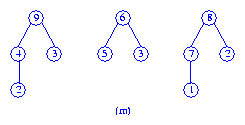In the second pass (which is a right to left pass), the two rightmost trees of Figure (m) are first melded to get the tree of Figure (n).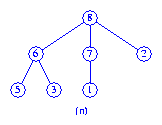Then the tree of Figure (m) with root `9` is melded with the tree of Figure (n) to get the final max tree which is shown in Figure (o).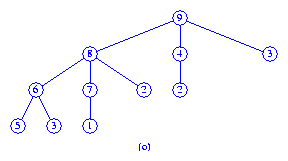Note that if the original pairing heap had `8` subtrees, then following the left to right melding pass we would be left with `4` max trees. In the right to left pass, we would first meld trees `3` and `4` to get tree `5`. Then trees `2` and `5` would be melded to get tree `6`. Finally, we would meld trees `1` and `6`.

In multi pass pairing heaps, the max trees that remain following the removal of the root are melded into a single max tree as follows:
1. Put the max trees onto a FIFO queue.
2. Extract two trees from the front of the queue, meld them and put the resulting tree at the end of the queue. Repeat this step until only one tree remains.

Consider the six trees of Figure (l) that result when the root of tree (k) is removed. First, we meld the trees with roots `9` and `4` and put the resulting max tree at the end of the queue. Next, the tree with roots `6` and `5` are melded and the resulting max tree is put at the end of the queue. And then, the tree with roots `7` and `8` are melded and the resulting max tree added to the queue end. The queue now contains the three max trees shown in Figure (m). Next, the max trees with roots `9` and `6` are melded and the result put at the end of the queue. We are now left with the two max trees shown in Figure (p).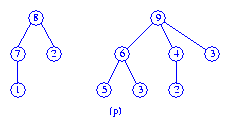Finally, the two max trees of Figure (p) are melded to get the max tree of Figure (q).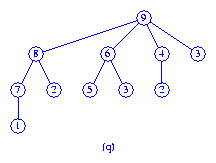remove
The operation `remove(theNode)` is handled as a `removeMax` operation when `theNode` is the root of the pairing heap. When `theNode` is not the tree root, the `remove` operation is done as follows:
1. Detach the subtree with root `theNode` from the tree.
2. Remove the node `theNode` and meld its subtrees into a single max tree using the two pass scheme if we are implementing a two pass pairing heap or the multi pass scheme if we are implementing a multi pass pairing heap.
3. Meld the max trees from steps 1 and 2 into a single max tree.

Implementation Considerations
Although we can implement a pairing heap using nodes that have a variable number of children fields, such an implementation is expensive because of the need to dynamically increase the number of children fields as needed. An efficient implementation is possible by using the binary tree representation of a tree (see Figure 12.15 of the text). Siblings in the original max tree are linked together using a doubly linked list. Each node has the three pointer fields `previous`, `next`, and `child`. The leftmost node in a doubly linked list of siblings uses its `previous` pointer to point to its parent. A leftmost child satisfies the property `x.previous.child = x`. The doubly linked list makes it is possible to remove an arbitrary element (as is required by the `remove` and `increaseKey` operations) in `O(1)` time.

Complexity and an Enhancement
You can verify that using the described binary tree representation, all pairing heap operations (other than `removeMax` and `remove`) can be done in `O(1)` time. The complexity of the `removeMax` and `remove` operations is `O(n)`, because the number of subtrees that have to be combined following the removal of a node is `O(n)`.

The paper The pairing heap: A new form of self-adjusting heap, Algorithmica, 1, March 1986, 111-129, by M. Fredman, R. Sedgewick, R. Sleator, and R. Tarjan, shows that the amortized complexity of the `put`, `meld`, `removeMax`, `remove`, and `increaseKey` operations is `O(log n)`, and that of the remaining operations is `O(1)`.

Experimental studies conducted by Stasko and Vitter reported in their paper Pairing heaps: Experiments and analysis, Communications of the ACM, 30, 3, 1987, 234-249 establish the superiority of two pass pairing heaps over multipass pairing heaps.

The above paper by Stasko and Vitter also proposes a variant of pairing heaps (called auxiliary two pass pairing heaps) that performs better than two pass pairing heaps. In an auxiliary two pass pairing heap we maintain a main max tree `mainTree`, an auxiliary list `auxList` of max trees, and a pointer `maxElement` to the max tree that has the largest root. Initially, the tree `mainTree`, the list `auxList`, and the pointer `maxElement` are all `null`. The `isEmpty`, `size`, and `getMax` operations are performed exactly as they are in an ordinary two pass pairing heap. A `put` is done by adding a single-node max tree to the end of the list `auxList` and updating the pointer `maxElement` if necessary. An `increaseKey(newValue, theNode)` is done by detaching the tree with root `theNode` from its parent, changing the key in `theNode` to `theValue`, updating `maxElement` if necessary, and adding the tree with root `theNode` to the end of the list `auxList`.

The `removeMax` operation of an auxiliary two pass pairing heap is done as follows:
1. Meld the max trees in `auxList` using the multi pass scheme. That is, put the trees in `auxList` onto a FIFO queue and pairwise meld from the front of this queue until only one tree remains in the queue. Note that the result of each meld is added to the end of the queue.
2. Meld the main tree and the tree that results from the pairwise melding of Step 1.
3. The root of the single max tree that remains is the max element. This root is removed and the subtrees are melded into a single max tree using the two pass scheme.

The steps for the `remove(theNode)` operation are:
1. If `theNode = maxElement`, then a `removeMax` (as described above for the auxiliary two pass pairing heap) is done.
2. If `theNode` is the root of a max tree `T`, then a `removeMax` (as described for a two pass pairing heap) is done on `T`.
3. Otherwise,
(a)
Detach the subtree with root `theNode` from its tree `U`.
(b)
Remove the node `theNode` and meld its subtrees into a single max tree using the two pass scheme.
(c)
Meld the max trees from steps (a) and (b) into a single max tree.
(d)
Leave the resulting tree in `auxList` if the tree `U` was initially there.

To meld two auxiliary two pass pairing heaps, we do the following
1. Concatenate the auxialiary lists of the two pairing heaps.
2. Add one of the two main trees to the end of the auxiliary list created in step 1.
3. The other main tree becomes the main tree for the result.
4. `maxElement` for the result is the larger of the max elements of the two pairing heaps being melded.

Although an auxiliary multi pass pairing heap is also defined by Stasko and Vitter, this version performs worse than the basic multi pass pairing heap.